## 使用TensorFlow识别交通标志 转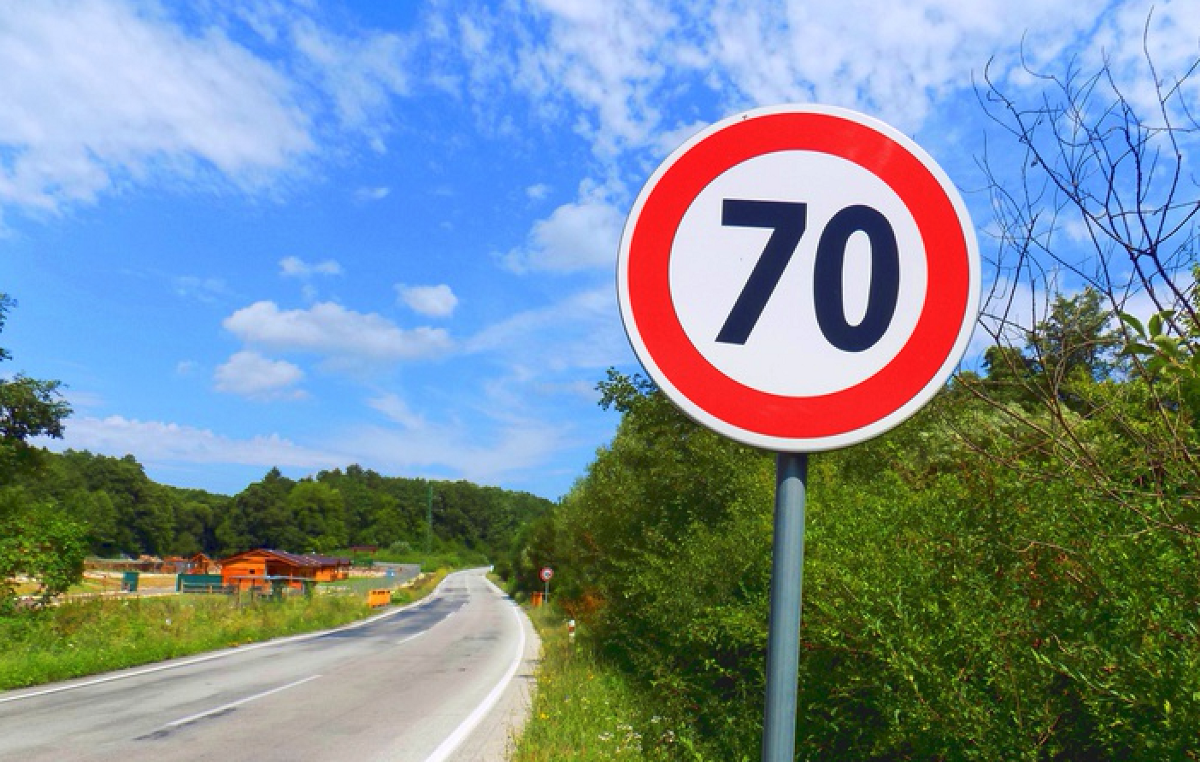# 运行代码

``docker run -it -p 8888:8888 -p 6006:6006 -v ~/traffic:/traffic waleedka/modern-deep-learning``

# 寻找训练数据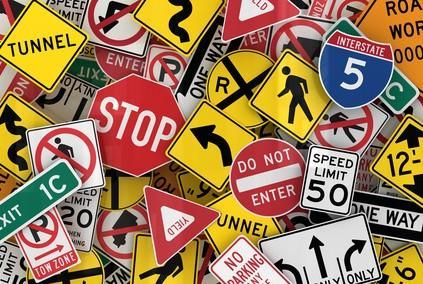• BelgiumTSC_Training (171.3MBytes)
• BelgiumTSC_Testing (76.5MBytes)

``````/traffic/datasets/BelgiumTS/Training/
/traffic/datasets/BelgiumTS/Testing/``````

# 探索数据集

``````def load_data(data_dir):
# Get all subdirectories of data_dir. Each represents a label.
directories = [d for d in os.listdir(data_dir)
if os.path.isdir(os.path.join(data_dir, d))]
# Loop through the label directories and collect the data in
# two lists, labels and images.
labels = []
images = []
for d in directories:
label_dir = os.path.join(data_dir, d)
file_names = [os.path.join(label_dir, f)
for f in os.listdir(label_dir)
if f.endswith(".ppm")]
for f in file_names:
images.append(skimage.data.imread(f))
labels.append(int(d))
return images, labels

images, labels = load_data(train_data_dir)``````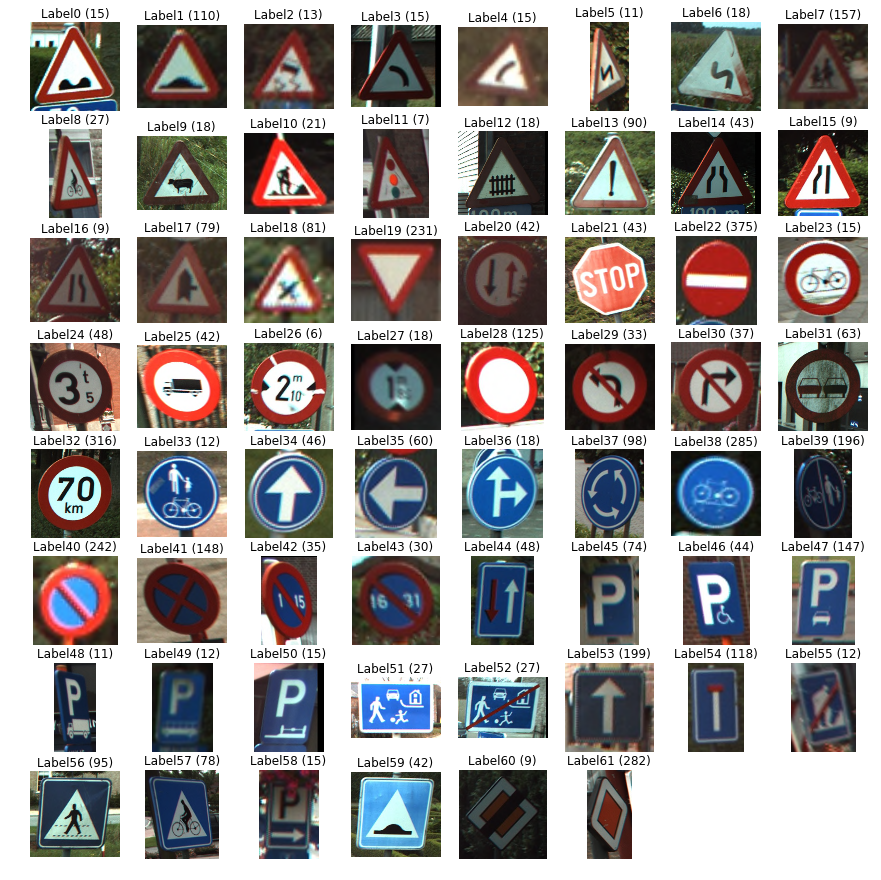The training set. consists of 62 classes. The numbers in parentheses are the count of images of each class.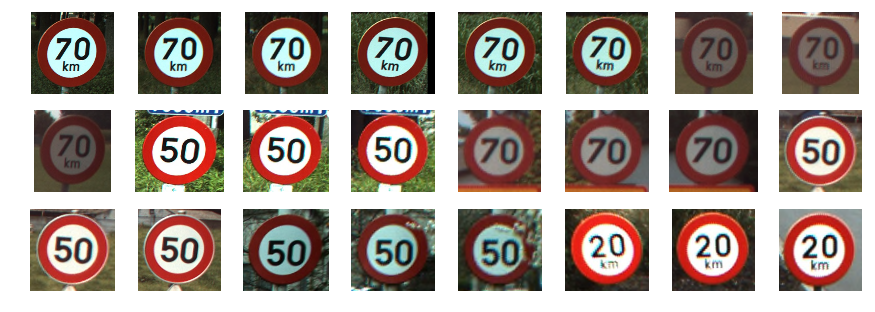Several sample images of label 32

# 处理不同尺寸的图片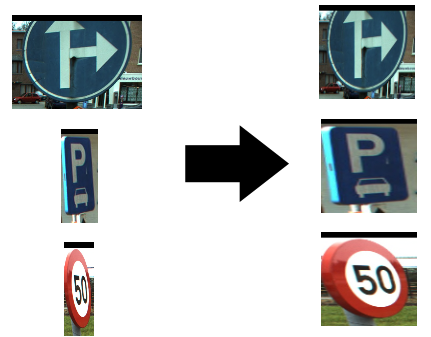Resizing images to a similar size and aspect ratio

``````for image in images[:5]:
print("shape: {0}, min: {1}, max: {2}".format(
image.shape, image.min(), image.max()))
Output:
shape: (141, 142, 3), min: 0, max: 255
shape: (120, 123, 3), min: 0, max: 255
shape: (105, 107, 3), min: 0, max: 255
shape: (94, 105, 3), min: 7, max: 255
shape: (128, 139, 3), min: 0, max: 255``````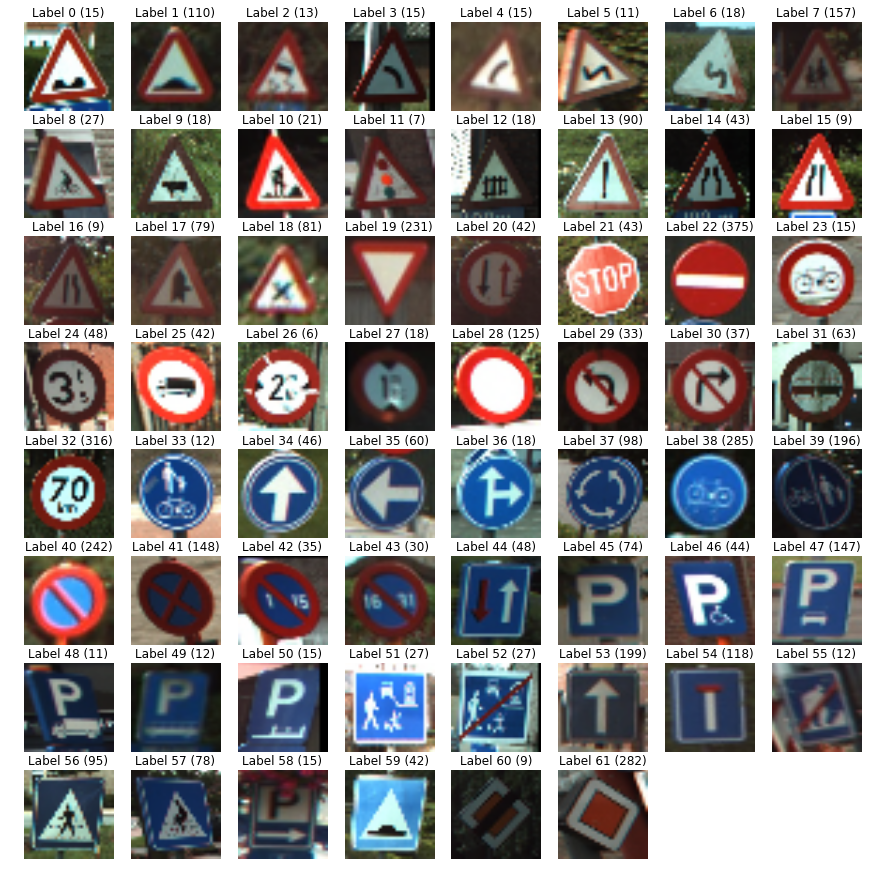Images resized to 32x32

# 最小可行模型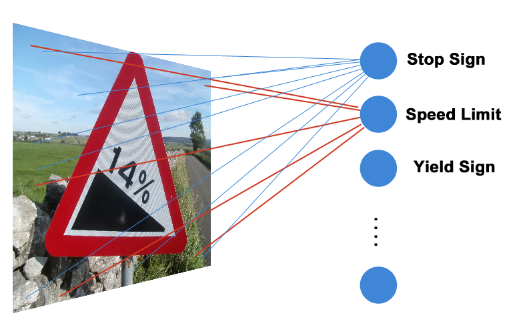``y = xW + b``

# 构建 TensorFlow 图

TensorFlow 在执行图中封装了神经网络的架构。构建的图包含了操作（简称为 Ops），比如 Add，Multiply，Reshape，..... 等等。这些操作在张量（多维数组）中对数据执行操作。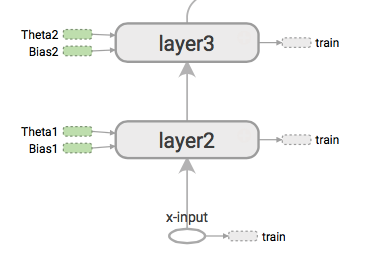Visualization of a part of a TensorFlow graph

``````# Create a graph to hold the model.
graph = tf.Graph()

# Create model in the graph.
with graph.as_default():
# Placeholders for inputs and labels.
images_ph = tf.placeholder(tf.float32, [None, 32, 32, 3])
labels_ph = tf.placeholder(tf.int32, [None])

# Flatten input from: [None, height, width, channels]
# To: [None, height * width * channels] == [None, 3072]
images_flat = tf.contrib.layers.flatten(images_ph)

# Fully connected layer.
# Generates logits of size [None, 62]
logits = tf.contrib.layers.fully_connected(images_flat, 62, tf.nn.relu)

# Convert logits to label indexes (int).
# Shape [None], which is a 1D vector of length == batch_size.
predicted_labels = tf.argmax(logits, 1)

# Define the loss function.
# Cross-entropy is a good choice for classification.
loss = tf.reduce_mean(tf.nn.sparse_softmax_cross_entropy_with_logits(
logits, labels_ph))

# Create training op.
train = tf.train.AdamOptimizer(learning_rate=0.001).minimize(loss)

# And, finally, an initialization op to execute before training.
# TODO: rename to tf.global_variables_initializer() on TF 0.12.
init = tf.initialize_all_variables()``````

``graph = tf.Graph()``

``````with graph.as_default():
images_ph = tf.placeholder(tf.float32, [None, 32, 32, 3])
labels_ph = tf.placeholder(tf.int32, [None])``````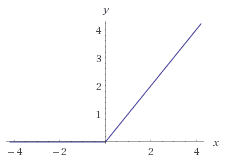The ReLU function

``f(x) = max(0, x)``

``````# Flatten input from: [None, height, width, channels]
# To: [None, height * width * channels] == [None, 3072]
images_flat = tf.contrib.layers.flatten(images_ph)
# Fully connected layer.
# Generates logits of size [None, 62]
logits = tf.contrib.layers.fully_connected(images_flat, 62,
tf.nn.relu)``````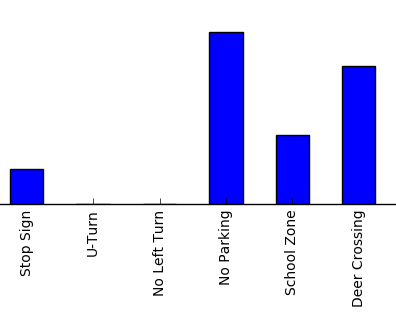Bar chart visualization of a logits vector

``````# Convert logits to label indexes.
# Shape [None], which is a 1D vector of length == batch_size.
predicted_labels = tf.argmax(logits, 1)``````

argmax 函数的输出结果将是一个整数，范围是 [0, 61]。

# 损失函数和梯度下降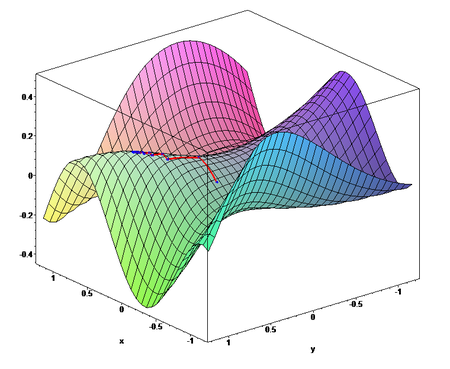Credit: Wikipedia

``````loss = tf.reduce_mean(
tf.nn.sparse_softmax_cross_entropy_with_logits(
logits, labels_ph))``````

``train = tf.train.AdamOptimizer(learning_rate=0.001).minimize(loss)``

``init = tf.initialize_all_variables()``

# 训练循环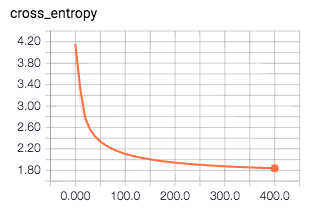``session = tf.Session(graph=graph)``

``session.run(init)``

``````for i in range(201):
_, loss_value = session.run(
[train, loss],
feed_dict={images_ph: images_a, labels_ph: labels_a})
if i % 10 == 0:
print("Loss: ", loss_value)``````

``````Loss:  4.2588
Loss:  2.88972
Loss:  2.42234
Loss:  2.20074
Loss:  2.06985
Loss:  1.98126
Loss:  1.91674
Loss:  1.86652
Loss:  1.82595
...``````

# 模型使用

``````# Pick 10 random images
sample_indexes = random.sample(range(len(images32)), 10)
sample_images = [images32[i] for i in sample_indexes]
sample_labels = [labels[i] for i in sample_indexes]
# Run the "predicted_labels" op.
predicted = session.run(predicted_labels,
{images_ph: sample_images})
print(sample_labels)
print(predicted)

Output:
[15, 22, 61, 44, 32, 22, 57, 38, 56, 38]
[14  22  61  44  32  22  56  38  56  38]``````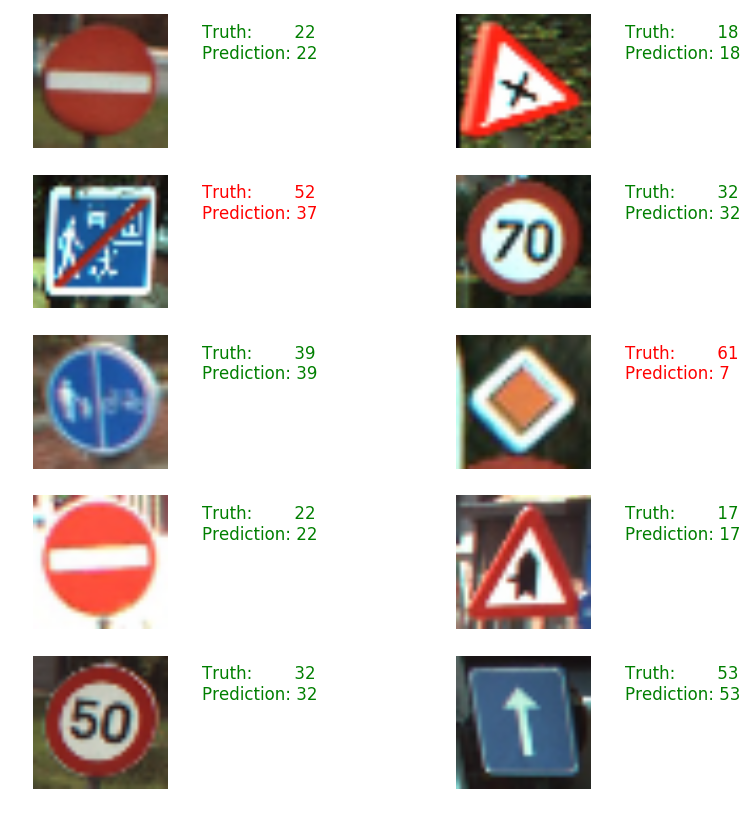# 评估

``````# Run predictions against the full test set.
predicted = session.run(predicted_labels,
feed_dict={images_ph: test_images32})
# Calculate how many matches we got.
match_count = sum([int(y == y_)
for y, y_ in zip(test_labels, predicted)])
accuracy = match_count / len(test_labels)
print("Accuracy: {:.3f}".format(accuracy))``````

# 关闭会话

``````# Close the session. This will destroy the trained model.
session.close()``````### AllenOR灵感

AI在汽车中的应用：实用深度学习

https://mp.weixin.qq.com/s/NIza8E5clC18eMF_4GMwDw 深度学习的“深度”层面源于输入层和输出层之间实现的隐含层数目，隐含层利用数学方法处理（筛选/卷积）各层之间的数据，从而得出最终结...

Terminator2050
2018/09/18
0
0

08/14
0
0
Tensorflow入门——卷积神经网络MNIST手写数字识别

Image source: unsplash.com by Paweł Czerwiński 之前的文章我们介绍了如何用单层和多的 全连接层神经网络识别手写数字，虽然识别率能够达到98%，但是由于全链接神经网络本身的局限性，其...

Hongtao洪滔
04/02
0
0

ismdeep
2017/12/24
14.4K
3
Artificial Intelligence Yourself

TensorFlow是谷歌基于DistBelief进行研发的第二代人工智能学习系统，其命名来源于本身的运行原理。Tensor（张量）意味着N维数组，Flow（流）意味着基于数据流图的计算，TensorFlow为张量从流...

2018/07/16
19
0

FAT_mt

6
0

7
0
tabel 中含有复选框的列 数据理解

1、el-ui中实现某一列为复选框 实现多选非常简单: 手动添加一个el-table-column，设type属性为selction即可； 2、@selection-change事件：选项发生勾选状态变化时触发该事件 <el-table @sel...

everthing

6
0
【技术分享】TestFlight测试的流程文档

qtb999

10
0

2019年8月6日，Spring 官方在其博客宣布，Spring Boot 1.x 停止维护，Spring Boot 1.x 生命周期正式结束。 其实早在2018年7月30号，Spring 官方就已经在博客进行过预告，Spring Boot 1.X 将维...

Java技术剑

18
0